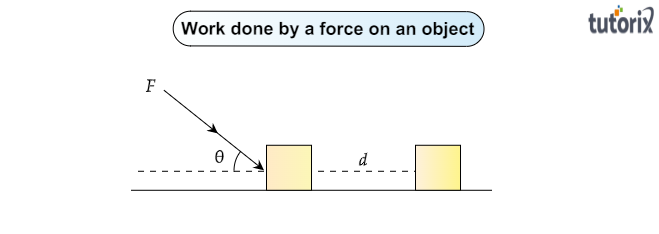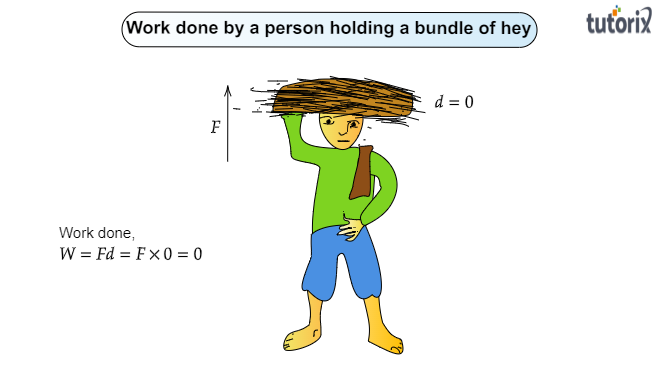# A person holds a bundle of hay over his head for 30 minutes and gets tired. Has he done some work or not? Justify your answer.

Given:

A person holds a bundle of hay over his head for 30 minutes and gets tired.

To do:

To find out whether he has done some work or not.

Solution:

To find out the work done by the person holding a bundle of hay over his head for 30 minutes let us know what is said to be the work done:

Work done:

When a force acts upon an object and the object is moved in a certain direction, it is said to be the work done by the force.Formula for the work done:

$\boxed{W=Fd\cos\theta}$

Here, $W\rightarrow$ work done

$F\rightarrow$ Force acted upon the object

$d\rightarrow$ displacement of the object

$\theta$ Angle between the force and the displacement

Based on the above definition of the work done and its formula we can easily find out the work done by the person holding a bundle of hay over his head for 30 minutes and getting tired.

Work done by the person:

When a person holds a bundle of hay over his head for 30 minutes and gets tired.As there is no displacement in the bundle of hay.

So displacement , $d=0$

Therefore, work done $W=Fd$

Or $W=F\times 0=0$

Therefore, the work done by the person is also zero.